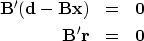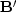Next: Differentiation by a complex Up: MULTIVARIATE LEAST SQUARES Previous: Inverse filter example

## Normal equations

The basic least-squares equations are often called the normal" equations. The word normal" means perpendicular. We can rewrite equation (16) to emphasize the perpendicularity. Bring both terms to the left, and recall the definition of the residualfrom equation (2):(23) (24)
Equation (24) says that the residual vectoris perpendicular to each row in thematrix. These rows are the fitting functions. Therefore, the residual, after it has been minimized, is perpendicular to the fitting functions.Next: Differentiation by a complex Up: MULTIVARIATE LEAST SQUARES Previous: Inverse filter example
Stanford Exploration Project
10/21/1998